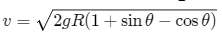# HC Verma Solutions Class 11 Chapter 8 Work and Energy

HC Verma Solutions Class 11 Chapter 8 Work and Energy gives students the opportunity to learn the right method of solving questions related to important topics like kinetic energy, potential energy, work done by the force of gravity,  finding power, etc. This chapter contains questions which are frequently asked in in prominent examinations such as JEE Advanced and students can develop better skills and prepare completely by referring to the solutions. You will get to learn topics of work and energy in the problems given here such as:

• We will be dealing with questions related to work done against resistance and work done against friction.
• Solve questions on the law of conservation of energy and the energy required to overcome friction.
• Understand problems related to work done when force is parallel to the inclined plane and tackle problems by applying the work-energy principle.

Students will further learn about topics like;

1. Kinetic Energy
2. Work and Work-energy Theorem
3. Calculation of Work Done
4. Work-energy Theorem for a System of Particles
5. Potential Energy
6. Conservative and Nonconservative Forces
7. Definition of Potential Energy and
8. Conservation of Mechanical Energy
9. Change in the Potential Energy in a Rigid-body-motion
10. Gravitational Potential Energy
11. Potential Energy of a Compressed or Exercises
12. Extended Spring
13. Different Forms of Energy: Mass Energy
14. Equivalence

## Class 11 Important Questions In Chapter 8

1. When a ball falls from a great height there are some changes to its gravitational potential energy. What happens to the energy when it reaches the ground? Also, explain what will happen to the energy after it strikes the ground?

2. Do you think that the work done by kinetic friction on an object have a result as Zero or positive?

3. You lift a heavy box from the ground and keep it on the storage shelf. Therefore, the work done by you on the box does not depend on

(a) the weight of the box (b) the time taken in completing the action (c) the path taken by the box (d) your own weight.

4. An object having certain mass moves down on a smooth vertical circular track. During the movement, the object is in

(a) radial equilibrium (b) horizontal equilibrium (c) vertical equilibrium (d) none of these.

5. A charged particle has a magnetic force that is always perpendicular to its velocity. Keeping this in mind can the magnetic force change the velocity and speed of the particle?

## Class 11 HC Verma Solutions Vol 1 Work and Energy Chapter 8

Question 1: The mass of cyclist together with the bike is 90 kg. Calculate the increase in kinetic energy if the speed increases from 60 km/h to 12 km/h.

Solution:

Initial speed = 6000 kmph = 5/3 m/sec

We know, K.E. = (1/2) mv2 = 1/2 x 90 x (5/3) 2 = 125 Joules

Final speed = 12 kmph = 10/3 m/s

K.E. = 1/2 x 90 x (10/3)2 = 500 Joules

Therefore, the increase in K.E. = 500 – 125 = 375 Joules

Question 2: A block of mass 2.00 kg moving at a speed of 10.0 m/s accelerates at 3.00 m/s2 for 5.00 s. Compute its final kinetic energy.

Solution:

Given, initial velocity u = 10m/sec, acceleration a= 3m/ and time t= 5sec

Find the final velocity at the end of 5 sec.

Therefore, final velocity = v = u + at

= 10 + 15 = 25 m/s

Now, Final K.E. = 1/2 mv2 = 1/2 x 2 x 252 = 625 Joules

Question 3: A box is pushed through 4.0 m across a floor offering 100 N resistance. How much work is done by the resisting force?

Solution:

Force = F = 100 N

S = 4m and θ = 0o

Now, ω = F.S = 100 x 4 = 400 Joules

Question 4: A block of mass 5.0 kg slides down an incline of inclination 30° and length 10 m. Find the work done by the force of gravity.

Solution:

Given, m = 5 kg, S = 10 m, θ = 300

We know, F = mg

Therefore, work done by the force of gravity = ω = mgh

= 5 x 9.8 x 5

= 245 J

Question 5: A constant force of 2.50 N accelerates a stationary particle of mass 15 g through a displacement of 2.50 m. Find the work done and the average power delivered.

Solution:

Given:

Displacement = S = 2.5m, m = 15g or 0.015 kg and F = 2.50N

Also, given the initial velocity u = 0, implies initial K.E =0

Acceleration = a = force/mass = 2.5/0.015 = 500/3 m

and v = 2500/3 m/s [using equation v2-u2=2as]

Now, Final K.E. = 1/2 mv2 = 1/2 x (0.015) x (2500/3) 2

= 25/4 J

Again, Work done = W = change in K.E. = 25/4 – 0 = 6.25 J

[Uisng work energy theorem]

Let us find the times taken for the displacement:

We know, v = u + at

[equation of uniform linear motion]

Thus, by substituting for v,u and a, we get t = 0.17sec

Now,

Power = P = Work/time = 6.25/0.17 = 36.08 watts or 36.1 watts.

Question 6: A particle moves from a r1 = (2m)i + (3m)j to another point r2 = (3m)i + (2m)j during which a certain force F = (5N)i + (5 N)j acts on it. Find the work done by the force on the particle during the displacement.

Solution:

Displacement of the particle = r = r1 – r2

= (2i + 3j) – (3i + 2j)

= -i + j

From given, work done W = F.r

=> W = ( 5i + 5j ) . (-i + j)

= (-5 + 5)

= o Joule

Question 7: A man moves on a straight horizontal road with a block of mass 2 kg in his hand. If he covers 40 m with an acceleration of 0.5 m/s2, find the work done by the man on the block during the motion.

Solution:

Mass of block = 2kg

acceleration = a = 0.5m/s2

Distance covered= s = 40m

Using, v2 – u2 = 2as

or v2 = u2 + 2as

Here u = 0

=> v2 = 40

Now by using the work energy theorem = W = ΔK.E.

= 1/2 mv2 – 1/2 mu2

On substituting the values, we get

W = 40 Joules

Question 8: A force F = a + bx acts on a particle in the x-direction, where a and b are constants. Find the work done by this force during a displacement from x = 0 to x = d.

Solution:

Given, F = a+bx

Here body moves in +ve x-direction from x=0 to x=d.

At x=0 => F=a and

At x=d => F’= a+bd

Average of forces at both the positions: (F+F’)/2

=> a + bd/2

Question 9: A block of mass 250 g slides down an incline of inclination 370 with a uniform speed. Find the work done against the friction as the block slides through 1.0 m.

Solution:

Mass = m = 0.25kg

displacement =s= 1m

Inclination=θ =37°

Also, g=10m/s2

Now,

Weight = mg= 0.25× 10=2.5N

The force against is the component of weight along the incline = mg sinθ = mg sin 37o

Work done against the friction force on the block = F× s i.e. mg sin 37 o = 1.50 J.

Question 10: A block of mass m is kept over another block of mass M and the system rests on a horizontal surface. A constant horizontal force F acting on the lower block produces an acceleration F/(2(m+M)) in the systems the two blocks always move together. (a) Find the coefficient of kinetic friction between the bigger block and the horizontal Surface. (b) Find the frictional force acting on the smaller block. (c) Find the work done by the force of friction on the smaller block by the bigger block during a displacement d of the system.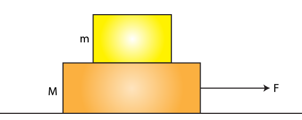Solution:

(a) Weight of the combined system = (m+M)g = N

acceleration =a = F/(2(M+m)) …(Given)

Frictional force = μ N = μ(m + M)g

By equating above equations, we have

F – μ (m + M)g = (m + M)a

on substituting the value of “a” and solving we have

μ = F/(2g(M+m))

(b) Let f be the frictional force acting on the smaller block

=> Force = mass x acceleration

= mF/(2(m+M))

(c)

velocity of the block can be evaluated by using uniform motion equation, v2 – u2 = 2as

When d is the displacement given.

Here u = 0

=> v = √[Fd/(m+M)]

And initial K.E =0

therefore, final K.E. = 1/2 mv2 = mFd/2(m+M)

Thus, So, work done by the frictional force on the smaller block by the larger block = change in kinetic energy = mFd/2(m+M)

Question 11: A box weighing 2000 N is to be slowly slid through 20 m on a straight track having friction coefficient 0.2 with the box. (a) Find the work done by the person pulling the box with a chain at an angle θ with the horizontal. (b) Find the work when the person has chosen a value of θ which ensures him the minimum magnitude of the force.

Solution: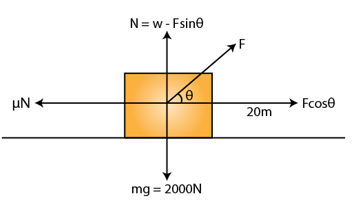Here g=acceleration due to gravity=10m/s2

w = weight of the box = mg = 2000 N

Force of friction = μN

From free body diagram of the box, N = w – Fsinθ

Now,

Fcosθ = μN = μ(w-Fsinθ)

F(cosθ + μsinθ) =μmg

or F = μmg/(cosθ + μsinθ)

(a)

Work done horizontally: W’ = F sinθ x 20

= μmg/(cosθ + μsinθ) x sinθ x 20

= 40000/(tanθ+5) Joules

Work done vertical= w” = 0 Joules

[as there is no displacement in the vertical direction]

Total work done on the box = W = W’ + W”

= 40000/(tanθ+5) + 0

= 40000/(tanθ+5) Joules

(b) Differentiate F w.r.t. θ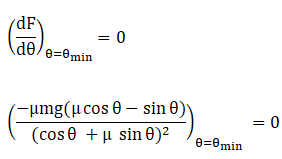Let θmin be the angle for which F is minimum.

=> μ cosθmin= sinθmin

=> tanθmin= μ

=> θmin = tan-1μ

Question 12: A block of weight 100 N is slowly slid up on a smooth incline of inclination 37o by a person. Calculate the work done by the person in moving the block through a distance of 2.0 m, if the driving force is (a) parallel to the incline and (b) in the horizontal direction.

Solution:

(a) Work done = w = Force along the line of displacement × displacement

=100 sin37° × 2

=120.363005

= 120 J (approx)

(b) Work done will be the same as above. This is so because the component of force along the line of displacement will still be the same i.e. 100 sin37°.

Question 13: Find the average frictional force needed to stop a car weighing 500 kg in a distance of 25 m if the initial speed is 72 km/h.

Solution:

m = 500 kg, u = 72 km/h or 20 m/s

s = 25 m

Using equation, v2 – u2 = 2as

or -a = (v2-u2)/2s

=> a = 400/50 = 8 m/s2

Now, friction force = f = ma = 500×8 = 4000N

Magnitude of frictional force required =4000N

Question 14: Find the average force needed to accelerate a car weighing 500 kg from rest to 72 km/h in a distance of 25 m.

Solution:

Speed of the car = 0 m/s

Accelerate the car to a speed of 72km/h or 20 m/s

K.E. = (1/2) ×500×20×20 = 105 J

The change in kinetic energy = 105 m/s

The work done in this process = change in kinetic energy

= 105-0 = 105 J

Also, work done = force × displacement

=> Force = work done/ displacement = 100000/25 = 4000 N

Question 15: A particle of mass m moves on a straight line with its velocity varying with the distance travelled according to the equation v = a√x, where a is a constant. Find the total work done by all the forces during a displacement from x = 0 to x = d.

Solution:

Given , v = a√x

Displacement = d

At x = 0 => v1 = 0 and at x = d => v2 = a√d

a = (v22 – v22)/2s = a2/2

Now, force = f = ma = ma2/2

Work done = w = Fs cos θ = ma2/2 x d = ma2d/2

Question 16: A block of mass 2.0 kg kept at rest on an inclined plane of inclination 37° is pulled up the plane by applying a constant force of 20 N parallel to the incline. The force acts for one second. (a) Show that the work done by the applied force does not exceed 40 J. (b) Find the work done by the force of gravity in that one second if the work done by the applied force is 40 J. (c) Find the kinetic energy of the block at the instant the force ceases to act. Take g = 10 m/s2.

Solution: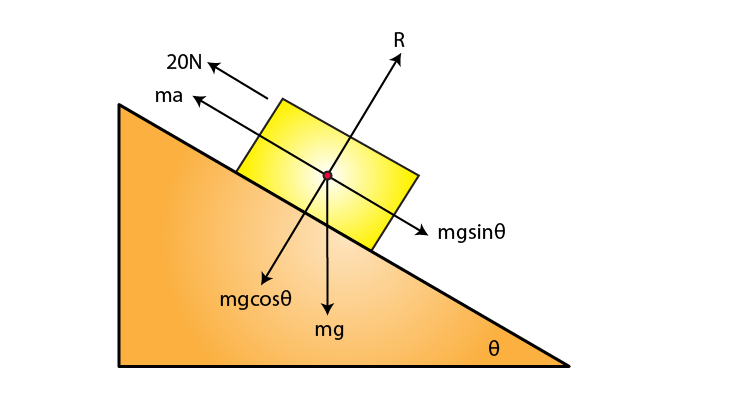mass = m = 2 kg

Time for which the force acts, t = 1 s

Force=F = 20 N

Acceleration due to gravity, g = 10 m/s2

Now, F = mg sinθ + ma

=> 20 = 2×10sin37° + 2×a

=> a = 4 m/s2

The distance travelled by the block = s = 1/2 at2

= 0.5×4×1×1

= 2m

(a) Maximum work done = Fs

= 20 × 2

= 40 J

(b) If the work done by the applied force is 40 J, then

Distance travelled by the block = work done/ force applied

= 40/20 = 2m

Height corresponding to this distance = h = 2sin37° = 1.2 m

Work done by force of gravity = -mgh

= -2 × 10 × 1.2

= -24 J

(-ve sign due to displacement is against gravity)

(c) Speed of the block after 1s = at = 4×1 = 4 m/s

Corresponding K.E. = 1/2 mv2

= 0.5 × 2 × 4 × 4

= 16 J

Question 17: A block of mass 2.0 kg is pushed down an inclined plane of inclination 37o with a force of 20 N acting parallel to the incline. It is found that the block moves on the incline with an acceleration of 10 m/s2. If the block started from rest, find the work done (a) by the applied force in the first second, (b) by the weight of the block in the first second and (c) by the frictional force acting on the block in the first second. Take g = 10 m/s2.

Solution: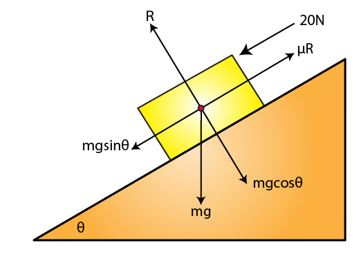m = 2kg

θ = 37°

F = 20N

Acceleration = a = 10 m/s2

(a) Find work done by the applied force in the first second

Here t = 1 sec

s = ut+ 1/2 x at2 = 5m

Work done = W = Fs cosθ = 20×5 = 100 J

(b) Work done by the weight of the box will be equal to the potential energy of the box.

W = Potential energy of the box = mass×g×height = mgh

= m×g×s×sin37°

=2×10×5×sin37°

= 60 J

(c) Frictional force = μR = mgsinθ

Work done by this force on the body = W = mgsinθ × s × cosΦ

W = 2×10×sin37°×s×cos180°

W = -60 J

Question 18: A 250 g block slides on a rough horizontal table. Find the work done by the frictional force in bringing the block to rest if it is initially moving at a speed of 40 cm/s. If the friction coefficient between the table and the block is 0.1, how far does the block move before coming to rest?

Solution: m = 250 g or 0.25 kg

u = 40cm/s or 0.4 m/s

Initial K.E. = mu2/2

= 0.25 × 0.4 × 0.4 × 0.5

= 0.02 J

Final speed of the block, v = 0 m/s

Final K.E. = mv2/2

= 0.25 × 0 × 0 × 0.5

= 0 J

Let “s m” distance the block moves before coming to rest

Coefficient of friction= μ = 0.1

Acceleration due to gravity, g=9.8 m/s2

Frictional force, F = μmg

= 0.1 × 0.25 × 9.8

= 0.245 N

Work done by the frictional force = Δ kinetic energy

W = 0-0.02

W = -0.02 J

Also, this work done by frictional force on the block = Fs cosθ

-0.02 = W = 0.245 × s × cos180°

-0.02 = -0.245s

s = 0.082 m or 8.2 cm

Which is the distance before the block stops due to the frictional force.

And work done by the frictional force on the block = -0.02 Joule

Question 19: Water falling from a 50 m high fall is to be used for generating electric energy. If 1.8 × 105 kg of water falls per hour and half the gravitational potential energy can be converted into electric energy, how many 100 W lamps can be lit?

Solution:

Height = h = 50 m and Mass = 1.8 × 105 kg

Mass of water falling per sec, m = (18×105)/(60×60) = 50 kg

Gravitational potential energy released per sec = mgh = 50 × 9.8 × 50 = 24500 J

Electrical energy generated per sec = (Gravitational potential energy)/2

= 24500/2 = 12250 J

Therefore, number of 100 W lamps that can be lit from this power = 12250/100 or 122 lamps(approx)

Question 20: A person is painting his house walls. He stands on a ladder with a bucket containing paint in one hand and a brush in other. Suddenly the bucket slips from his hand and falls down on the floor. If the bucket with the paint had a mass of 6.0 kg and was at a height of 2.0 m at the time it slipped, how much gravitational potential energy is lost together with the paint?

Solution:

Mass = m = 6.0 kg

Initial height = 2.0 m

Final height = 0.0 m

Acceleration due to gravity, g = 9.8 m/s2

Change in potential energy of the bucket = mg(final height – initial height)

= 6.0 × 9.8 × (0.0-2.0)

= -117.6 J

Loss in gravitational potential energy = 117.6 J = 118 J (approx.)

Question 21: A projectile is fired from the top of a 40 m high chit with an initial speed of 50 m/s at an unknown angle. Find its speed when it hits the ground.

Solution:

Height = h = 40 m

Initial speed = u = 50 m/s

Final speed = v m/s

Acceleration due to gravity, g = 10 m/s2

We know, v2 – u2 = 2gh [equation of motion]

or v2 = u2 + 2gh

=> v2 = 502 + 2×10×40

=> v2 = 3300

=> v = 57.45 m/s

Question 22: The 200 m free style women’s swimming gold medal at Seol Olympic 1988 went to Heike Friendrich of East Germany when she set a new Olympic record of 1 minute and 57.56 seconds. Assume that she covered most of the distance with a uniform speed and had to exert 460 W to maintain her speed. Calculate the average force of resistance offered by the water during the swim.

Solution:

Distance covered = d= 200 m

Time in which she covered the 200m distance = t = 1 minute 57.56 seconds or 117.56 seconds

Power = 460 W or J/s

Work done = W = Power x Time

= 460 x 117.56

= 54077.6 J

Work done = W = Force × distance covered

Magnitude of this force of resistance = W/d

= 54077.6/200

= 270.388 N = 270 N (approx)

Question 23: The US athlete Florence Griffith-Joyner won the 100 m sprint gold medal at Seol Olympic 1988 setting a new Olympic record of 10.54 s. Assume that she achieved her maximum speed in a very short-time and then ran the race with that speed till she crossed the line. Take her mass to be 50 kg. (a) Calculate the kinetic energy of Griffith-Joyner at her full speed. (b) Assuming that the track, the wind etc. offered an average resistance of one tenth of her weight, calculate the work done by the resistance during the run. (c) What power Griffith-Joyner had to exert to maintain uniform speed?

Solution:

m = 50 kg

v = 100/10.54 = 9.48766603 or 9.4877 m/s

(a) Kinetic energy of Griffith-Joyner at this speed = 1/2 mv2

= 50×9.4877×9.4877×0.5

= 2250.411 or 2250 J

(b) Force of resistance= F = weight of the athlete / 10

= 50 × 9.8 × 0.1

= 49 N

Her, acceleration a = 0 m/s2

=> Force in the direction of motion =F = ma = 0

Total work done by resistance = Fscosθ

= 49 × 100 × cos 180°

= -4900 J

(c) Power = work done / time for which the work is done

= 4900/10.54

= 464.89

= 465 W (approx)

Question 24 : A water pump lifts water from a level 10 m below the ground. Water is pumped at a rate of 30 kg/minute with negligible velocity. Calculate the minimum horsepower the engine should have to do this.

Solution:

h = 10 m

Flow Rate = 30 kg/minute or 0.5 kg per sec

Now,

Power = Work done/Time

= [mass × acceleration due to gravity × height]/ time = mgh/t

= 0.5 × 9.8 × 10

= 49 W

So, horse power = Power/746 = 49/746 horsepower = 6.6×10-2 hp

Question 25: An unruly demonstrator lifts a stone of mass 200 g from the ground and throws it at his opponent. At the time of projection, the stone is 150 cm above the ground and has a speed of 3.00 m/s. Calculate the work done by the demonstrator during the process. If it takes one second for the demonstrator to lift the stone and throw, what horsepower does he use?

Solution:

Mass = m = 200 g = 0.2 kg

h = 150 cm = 1.5 m

v = 3 m/s and t = 1 sec

Total Work done = Kinetic energy + Potential energy

= 1/2 × mv2 + mgh

= 0.5 ×0.2 × 32 + 0.2 × 9.8 × 1.5

= 3.84 J

Power = Work done/ time

= 3.84/1

= 3.84 W

Horsepower used = 3.84/746 hp = 5.14 × 10-3 hp

Question 26: In a factory it is desired to lift 2000 kg of metal through a distance of 12 m in 1 minute. Find the minimum horsepower of the engine to be used.

Solution:

Mass = m = 2000 kg

Height = h = 12 m

Time = t = 1 min or 60 s

So, power = Work done in lifting/Time taken

= mgh/t

= (2000×10×12)/60

= 3920 W

= 3920/746 hp

= 5.3 hp (approx)

Question 27: A scooter company gives the following specifications about its product.

Weight of the scooter — 95 kg

Maximum speed — 60 km/h

Maximum engine power — 3.5 hp

Pick up time to get the maximum speed — 5 s

Check the validity of these specifications.

Solution:

m = 95 kg

Maximum speed=v = 60 km/h or 50/3 m/s

t = 5 s

Engine power required to achieve this speed in given time = mv2/2t

= 95 x (50/3)2 x 1/10

= 3.537 hp

little higher than the given maximum power.

An engine of 3.5 hp can not exactly produce 60 km/h speed in 5 s. Hence, these specifications are somewhat over claimed.

Question 28: A block of mass 30.0 kg is being brought down by a chain. If the block acquires a speed of 40.0 cm/s in dropping down 2.00 m, find the work done by the chain during the process.

Solution:

Here, s = 2 m, m = 30 kg and u = 40 cm/s = 0.4 m/s

Final speed of the block = v = 0 m/s

Work done on the block = change in kinetic energy of the block

= 1/2 × m × (v2 – u2)

= 0.5 × 30 × [0 – (0.4×0.4)]

= -2.4 J

Let T be the tension produced in the string.

Net force on the block = Tension in the string – Weight of the block

F = T – mg

= T – 30 × 9.8

= T – 294

Work done on the block= W = Fs = (T-294) x 2

-2.4 = (T-294) × 2

T = 292.8 N

Work done on the block = Ts cos180°

= 292.8 × 2 × -1

= -586 J (approx)

Question 29: The heavier block in an Atwood machine has a mass twice that of the lighter one. The tension in the string is 16.0 N when the system is set into motion. Find the decrease in the gravitational potential energy during the first second after the system is released from rest.

Solution: Mass of heavier block = 2m

Tension in the string, T = 16 N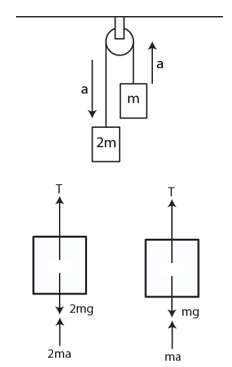from the free body diagram,

T – 2mg + 2ma = 0 …(1)

T – mg – ma = 0 …(2)

From (1) and (2)

T = 4 ma

=> a = 16/4m = (4/m) m/s2

Again, s = ut + 1/2 at2

=> s = 2/m

[Here u = 0]

Now, net mass = 2m – m = m

Decrease in P.E. = mgh = mg x 2/m = 19.6 J

[using g = 10m/s2]

Question 30: The two blocks in an Atwood machine have masses 2.0 kg and 3.0 kg. Find the work done by gravity during the fourth second after the system is released from rest.

Solution:

Mass of the heavier block = m1 = 3 kg

Mass of the lighter block = m2 = 2 kg

Let the tension in the string be T and acceleration in the system be a.

and t = during the 4th second

from the free body diagram,

T – 3g + 3a = 0 …(1)

T – 2g – 2a = 0 …(2)

From (1) and (2)

a = g/5 m/s2

Distance travelled in t sec,

st = a/2 (2n – 1) = 6.86 m

[Here n = 4 and g = 9.8 m/s2]

Now,

Work done by gravity = (m2 – m1)×g×h

= (3-2) × 9.8 × 6.86

= 67 N (approx)

Question 31: Consider the situation shown in figure (below). The system is released from rest and the block of mass 1.0 kg is found to have a speed 0.3 m/s after it has descended through a distance of 1 m. Find the coefficient of kinetic friction between the block and the table.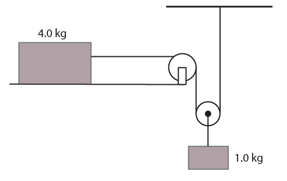Solution:

Mass of the block on the table = m1 = 4 kg

Mass of the block hanging from the table = m2 = 1 kg

Initial speed = u = 0 m/s

Distance travelled = s = 1 m

Speed at a later time = v = 0.3 m/s

using equation, 2as = v2 – u2

=> 2×a×1 = 0.3×0.3 – 0

=> a = 0.045 m/s2

Let 2T be the tension in the string.

m2a = m2g – 2T

2T = 1×(0.045 + 9.8)

T = 4.9225 N

Let the force of friction F and tension in the string will be T for the 4kg block on the table

T – F = 2m1a

Now, F = μm1g

Where, μ = Coefficient of friction between the block and the table

T – μm1g = 2m1a

4×μ×9.8 = 4.9225 – 2×4×0.045

or μ = 0.12 (approx)

Question 32: A block of mass 100 g is moved with a speed of 5.0 m/s at the highest point in a closed circular tube of radius 10 cm kept in a vertical plane. The cross-section of the tube is such that the block just fits in it. The block makes several oscillations inside the tube and finally stops at the lowest point. Find the work done by the tube on the block during the process.

Solution:

mass = m = 100 g or 0.1 kg

h = diameter of the block = 2×10 = 20 cm or 0.2 m

Work done by gravity = W = -mgh

= 0.1 × 9.8 × 0.2

= 0.196 J

Initial kinetic energy = 1/2 × m v2

= 0.5 × 0.1 × 52

= 1.25 J

Final kinetic energy = 0 J

Change in kinetic energy of the block = 0 – 1.25 = -1.25 J

Now,

Work done by the tube = change in kinetic energy – work done by gravity

= -1.25 – 0.196

= -1.45 J

Question 33: A car weighing 1400 kg is moving at a speed of 54 km/h up a hill when the motor stops. If it is just able to reach the destination which is at a height of 10 m above the point, calculate the work done against friction (negative of the work done by the friction).

Solution:

Mass =m = 1400 kg

Height = h = 10 m

Work done by gravity = -mgh

= -1400×9.8×10

= -137200

Initial speed = 54 km/h = 15 m/s

Initial kinetic energy = 1/2 × m v2

= 0.5 × 1400 × 15 × 15

= 157500 J

Final kinetic energy = 0 m/s

Change in kinetic energy of the car = 0 – 157500 = – 157500 J

Also, Work done againest friction = Change in kinetic energy – work done by gravity

= -157500 – (-137200)

= -157500 + 137200

= -20300 J

Therefore, Work done against friction = 20300 J

Question 34: A small block of mass 200 g is kept at the top of a frictionless incline which is 10 m long and 32 in high. How much work was required (a) to lift the block from the ground and put it at the top, (b) to slide the block up the incline? What will be the speed of the block when it reaches the ground, if (c) it falls off the incline and drops vertically on the ground (d) it slides down the incline? Take g = 10 m/s2.

Solution:

Mass = m = 200 g = 0.2 kg

Height of the incline plane = h = 3.2 m

Length of the incline plane = L = 10 m

Acceleration due to gravity, g = 10 m/s2

(a) Required work for lifting the block to the top of the incline = mgh

= 0.2×10×3.2

= 6.4 J

(b) Work done against friction = 0 J

Required work for sliding the block up = Work done against gravity

= 6.4 J

(c) Speed of the block when it is at rest on the top, u = 0 m/s

According to equation of motion, v2 – u2 = 2gh

=> v2 – 0 = 2×10×3.2

=> v = 8 m/s

(d) When the block slides down,

Change in KE = work done by gravity + work done by friction

=> 1/2 mv2 = mgh + 0

=> v2 = 2×10×3.2 = 64

or v = 8 m/s

Question 35: In a children’s park, there is a slide which has a total length of 10 m and a height of 8.0 m (below figure). Vertical ladder are provided to reach the top. A boy weighing 200 N climbs up the ladder to the top of the slide and slides down to the ground, The average friction offered by the slide is three tenth of his weight. Find (a) the work done by the boy on the ladder as he goes up, (b) the work done by the slide on the boy as he comes down (c) the work done by forces inside the body by the boy.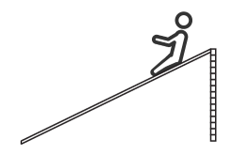Solution:

(a) Work done by the boy on the ladder as he goes up = 0 J

[As he goes against gravity]

(b) Weight = 200 N

Frictional force = (3/10) of the weight of the boy

= 60 N

Work done = Frictional force × Length of the ladder × cosθ

= 60 × 10 × cos180°

= -600 J

(c) the work done by forces inside the body by the boy.

Work done against gravity = Weight of the boy × height of the ladder

= 200 × 8

= 1600 J

Question 36: Figure (Below) shows a particle sliding on a frictionless track which terminates in a straight horizontal section. If the particle starts slipping from the point A, how far away from the track will the particle hit the ground?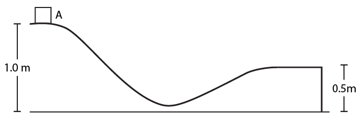Solution:

Let m be the mass of the particle.

Acceleration due to gravity = g = 9.8 m/s2

The height of the particle at point A = h = 1.0 m (Given)

The height of the particle at point where it terminates into straight horizontal section, h= 0.5 m (Given)

Now, total energy:

(Kinetic energy)_initial + (Potential energy)_initial = (Kinetic energy)_final + (Potential energy)_final

=> 0 + mgh at A = (1/2) mv2 + mgh at point of termination

=> m×9.8×1 = 0.5×m×v2 + m×9.8×0.5

=> 9.8 = 0.5v2 + 4.9

=> v = 3.13 m/s

The particle starts sliding from rest, from the equation of motion

=> h = 1/2 gt2

=> 0.5 = 0.5 × 9.8 × t2

=> t = 0.32 s

Which is the time taken by particle to reach the termination point .

Again, The horizontal distance that the particle will travel = speed × time

= 3.13 × 0.32

=1 m (approx)

Question 37: A block weighing 10 N travels down a smooth curved track AB joined to a rough horizontal surface (figure below). The rough surface has a friction coefficient of 0.20 with the block. If the block starts slipping on the track from a point 1.0 m above the horizontal surface, how far will it move on the rough surface?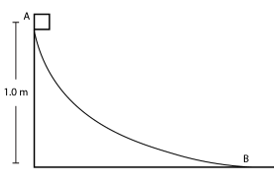Solution:

Coefficient of friction = μ = 0.20

Height of the point A = h = 1.0 m

Weight of the block = 10 N

Frictional force = F = μ × weight of the block

= 0.20 × 10

= 2 N

Let s be the displacement of the box from point A.

Loss in potential energy = Work done by the frictional force = Fs

Weight of the block × h = 2 × s

10 × 1 = 2 × s

s = 5 m

Thus, the block will move 5 m before stopping on the rough surface.

Question 38: A uniform chain of mass m and length l overhangs a table with its two third part on the table. Find the work to be done by a person to put the hanging part back on the table.

Solution:

Mass of the part of chain on the table = 2m/3 (given)

=> Mass of the part hanging from the table = m – 2m/3 = m/3

So, length of the part of chain = 2l/3

Length of the part of chain hanging from the table = l/3

=> Centre of the mass m/3 will be at l/6

Potential energy = m/3 x g x 1/6 = mgl/18

Therefore, Work done to put the hanging part back on table is mgl/18

Question 39: A uniform chain of length L and mass M overhangs a horizontal table with its two third part on the table. The friction coefficient between the table and the chain is μ. Find the work done by the friction during the period the chain slips off the table.

Solution:

A uniform chain of length L and mass M overhangs a horizontal table with its two third part on the table. (Given)

small element of the chain having length dx and mass dM.

then small mass will be dM = m/L dx

Force of friction = dF = μ g dM = μ g m/L dx

Now,

Work done on this small element by friction =

dW = dF . x = μ g m/L dx

Where x is the displacement of the small element by friction.

Again,

Total work done = W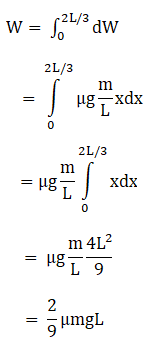Question 40: A block of mass 1 kg is placed at the point A of a rough track shown in figure (below). If slightly pushed towards right, it stops at the point B of the track. Calculate the work done by the frictional force on the block during its transit from A to B.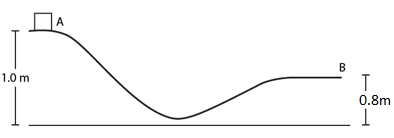Solution:

Work done by the frictional force = Change in potential energy of block

= Mass × acceleration due to gravity × change in height

= 1 × 10 × (0.8 – 1)

= –2J

Question 41: A block of mass 5.0 kg is suspended from the end of a vertical spring which is stretched by 10 cm under the load of the block. The block is given a sharp impulse from below so that it acquires an upward speed of 2.0 m/s. How high will it rise? Take g = 10 m/s2.

Solution:

Let h be the height to which the block will rise.

Mass of the block, m = 5 kg

Upward speed of the block = v = 2 m/s

Now, mgh = 1/2 mv2

=> h = 0.2 m

Therefore, The block will rise up to 0.2 m or 20 cm.

Question 42: A block of mass 250 g is kept on a vertical spring of spring constant 100 N/m fixed from below. The spring is now compressed to have a length 10 cm shorter than its natural length and the system is released from this position. How high does the block rise? Take g = 10 m/s2.

Solution:

Mass of the block = 250 g or 0.250 kg (Given)

A block of mass 250 g is kept on a vertical spring of spring constant 100 N/m (Given)

Height of the block rising is 20 cm

gravity due to acceleration = g = 10 m/s2.

We know, formula of the energy stored in spring compression:

Energy = 1/2 kx2

and energy stored in the block

Energy’ = mgh

Applying the conservation of the mass, between mass of block and spring compression we have the height,

mgh = 1/2 kx2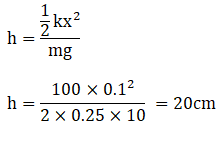The required height is 20 cm.

Question 43: Figure (Below) shows a spring fixed at the bottom end of an incline of inclination 37°. A small block of mass 2 kg starts slipping down the incline from a point 4.8 m away from the spring. The block compresses the spring by 20 cm, stops momentarily and then rebounds through a distance of 1 m up the incline. Find (a) the friction coefficient between the plane and the block and (b) the spring constant of the spring. Take g = 10 m/s2.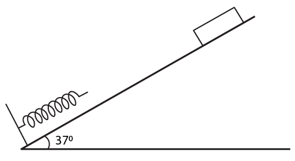Solution:

coefficient of friction = 0.5 and the spring constant = k = 1000 N/m

angle of inclination of the plane = 37o

Find energy stored in spring compression:

Energy = (1/2 ) kx2

And, energy stored in the block:

Energy’ = μ mgh

By applying, conservation of energy we get

mg sin 37o h = μ Rh + 1/2 kx2

[The block on the inclined plane is derived in two forms of derivatives with vertical mg sinθ and horizontal mg cosθ].

=> mg sin 37o (0.2+4.8) = μ R(0.2+4.8) + 1/2 kx2

=> 60 – 80μ = 0.02k …(i)

Again, For the upward return motion

mg sin 37o (1) = μ R(1) + 1/2 kx2

=> 12 – 16μ = 0.02k …(ii)

μ = 0.5

(i) => k = 1000 N/m

Question 44: A block of mass m moving at a speed u compresses a spring through a distance x before its speed is halved. Find the spring constant of the spring.

Solution:

A block of mass m moving at a speed u compresses a spring through a distance x before its speed is halved. (Given)

Find energy stored in spring compression:

Energy = 1/2 kx2

And, energy stored in the block:

Energy’ = (1/2) mu2

The velocity of the block “u” becomes u/2 after compression, making the initial kinetic energy as

K = (1/2) mu2

The total energy or the initial kinetic energy is equal to final kinetic energy and the spring compression energy.

=> (1/2) mu2 = (1/2) m(u/2) 2 + (1/2) kx2

=> kx2 = m(3/4 u2)

or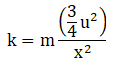Which is the spring constant.

Question 45: Consider the situation shown in figure(below). Initially the spring is upstretched when the system is released from rest. Assuming no friction in the pulley, find the maximum elongation of the spring.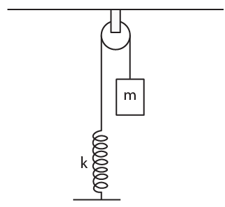Solution:

Find energy stored in spring compression:

Energy = 1/2 kx2

And, energy stored in the block:

Energy’ = mgh

In the potential energy of the block is given as x, equating the potential energy with the spring energy is

(1/2) kx2 = mgh

if h = x

=> (1/2) kx2 = mgx

=> x = 2mg/k

The maximum elongation of the spring is x = 2mg/k

Question 46: A block of mass m is attached to two upstretched springs of spring constants k1 and k2 as shown in figure (below). The block is displaced towards right through a distance x and is released. Find the speed of the block as it passes through the mean position shown.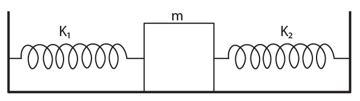Solution:

A block of mass “m” is attached with two spring constants k1 and k2 and the block is released on the right.

Energy stored in spring compression:

Energy = (1/2) kx2

Where, value of spring constant = k, the compression distance = x.

And, energy stored in the block:

Energy’ = (1/2) mv2

Where, the mass of the block = m, and velocity of the block = v

The conservation of the energy between spring energy and potential energy is given as

(1/2 ) k1 x2 + (1/2) k2 x2 = (1/2) mv2

mv2 = x2 (k1 + k2)

or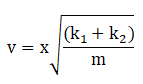Which is the speed of the block.

Question 47: A block of mass m sliding on a smooth horizontal surface with a velocity (vector v) meets a long horizontal spring fixed at one end and having spring constant k as shown in figure (below). Find the maximum compression of the spring. Will the velocity of the block be the same as (vector v) when it comes back to the original position shown?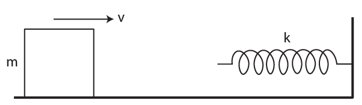Solution:

The sliding velocity [vector v] of the block with spring constant k. (Given)

Energy stored in spring compression:

Energy = (1/2) kx2

Where, spring constant = k, the compression distance = x.

And, energy stored in the block:

Energy’ = (1/2) mv2

Where, the mass of the block = m, and velocity of the block = v

Also, The kinetic energy of the block = K = (1/2) mv2

On equating the energies together, we get the compression distance as

(1/2) kx2 = (1/2) mv2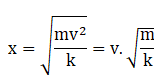Which is compression distance of the spring.

Question 48: A small block of mass 100 g is pressed against a horizontal spring fixed at one end to compress the spring through 5.0 cm (figure below). The spring constant is 100 N/m. When released, the block moves horizontally till it leaves the spring. Where will it hit the ground 2 m below the spring?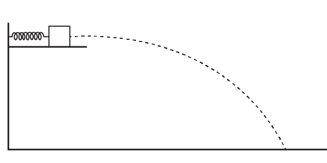Solution:

The block of mass = 100 g

Compressing the spring about 5 cm.

The height of the spring is 2 cm.

The spring constant = k = 100N/m.

Energy stored in spring compression:

Energy = (1/2) kx2

Where, spring constant = k, the compression distance = x.

And, energy stored in the block:

Energy’ = (1/2) mv2

Where, the mass of the block = m, and velocity of the block = v

Stored spring compression energy is calculated as

Energy = (1/2) kx2= 1/2 (100) (0.05)2 = 0.125 Joules

After the expansion of the spring, the compression energy turns into kinetic energy.

0.125 = (1/2) mv2

0.125 = 1/2 x (0.1)v2

or v = 1.58 m/s

Let’s evaluate time taken for the block to touch the ground:

s = ut + 1.2 gt2

2 = ½ x (9.8)t2 [Here u = 0]

or t = 0.63 sec

Horizontal distance covered by the block = S = ut = 1.58 x 0.63 = 1 m

Question 49: A small heavy block is attached to the lower end of a light rod of length l which can be rotated about its clamped upper end. What minimum horizontal velocity should the block be given so that it moves in a complete vertical circle?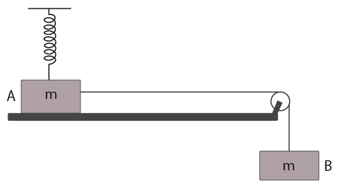Solution:

Let m is the mass of the object, v is the velocity, and assume that the lower end the potential energy of the block is zero.

Also g is the acceleration due to gravity and h is the height at which the object is kept.

Now, total energy = (1/2 ) mv2 + 0

At the maximum point the potential energy = (mg)2l.

By law of conservation of energy, we have

=> (1/2 ) mv2 = mg x 2l

or v = 2√(gl), is the minimum horizontal velocity.

Question 50: Figure (below) shows two blocks A and B, each having a mass of 320 g connected by a light string passing over a smooth light pulley. The horizontal surface on which the block A can slide is smooth. The block A is attached to a spring of spring constant 40 N/m whose other end is fixed to a support 40 cm above the horizontal surface. Initially, the spring is vertical and unstretched when the system is released to move. Find the velocity of the block A at the instant it breaks off the surface below it. Take g = 10 m/s2.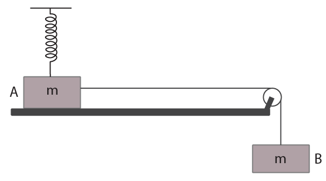Solution:

The masses of the blocks = 320 g

Spring constant = 40 N/m

Block B is attached at a height of 40cm from the horizontal surface, with a gravity of 10 m/s2.

We know, T = kx and T = mg

where, T = tension, k = spring constant, m = mass, x = compression distance and g = gravity.

Force/tension, applied on the block A due to spring is given as,

T = kx …(1)

where x = h(coseθ – 1)

By equating the y-axis direction of the T=mg force is

T sinθ = mg …(2)

Placing the T as kh(coseθ – 1), we get

(2)=> kh(coseθ – 1)sinθ = mg

0.4 x 40(coseθ – 1)sinθ = 0.32 x 9.8

sinθ = 0.8

Again,

(1) => kh(coseθ – 1) = kx

x = 0.1 m

Now, the difference in Kinetic Energy is equivalent to the force applied on the block.

v2 = gs – (1/2) kx2

= gh cotθ – (1/2) kx2

= 3 – 0.2/0.32

= 2.375

or v = 1.54 m/s, which is velocity of the block A.

Question 51: One end of a spring of natural length h and spring constant k is fixed at the ground and the other is fitted with a smooth ring of mass m which is allowed to slide on a horizontal rod fixed at a height h (figure below). Initially, the spring makes an angle of 37o with the vertical when the system is released from rest. Find the speed of the ring when the spring becomes vertical.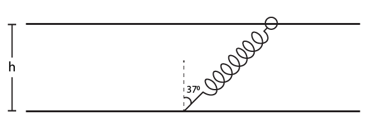Solution:

The spring with a spring constant = k, and at a height of h forming at an angle of 37 o

The conservation equation using conservation of static and dynamic energy such as spring energy and kinetic energy.

(½) mv2 = (1/2) kx2

Where, m = mass of the object, v = velocity, k = spring constant and x = elongation distance.

Now, speed of the block moved from the resting position

(1/2) mv2 = (1/2) kx2

or v = x √(k/m)

Again, height, h, is formed at angle 37o

h cos 37 o – h = x

and, if height of the horizontal plane and block as h’ = h/4, we get

v = (h/4) √(k/m), which is the velocity of the block.

Question 52: Figure (below) shows a tight rod of length l rigidly attached to a small heavy block at one end and a hook at the other end. The system is released from rest with the rod in a horizontal position. There is a fixed smooth ring at a depth h below the initial position of the hook and the hook gets into the ring as it reaches there. What should be the minimum value of h so that the block moves in a complete circle about the ring?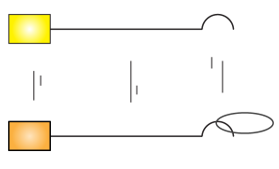Solution:

We are given that, the length of the tight rod is given as “l”, the gap between the final and initial position of the ring is given as “h”

The total energy in terms of kinetic and potential energy:

Total energy = ET =( ½) mv2 + mgl …(1)

Where, m = mass of the object, l = length of the object and g = acceleration in terms of gravity.

Also, the potential energy of the block = Ep = mgh …(2)

Equating (1) and (2), to find the gap of the final and initial position of the ring that is “h”.

(½) mv2 + mgl = mgh

or h = v2/2g + l

At v = 0, we have

h = l, which is the length of the height.

Question 53: The bob of a pendulum at rest is given a sharp hit to impart a horizontal velocity √(10 gl) where l is the length of the pendulum. Find the tension in the string when (a) the string is horizontal,

(b) the bob is at its highest point and

(c) the string makes an angle of 60o with the upward vertical.

Solution:

Equation of motion for the particle:

T – mg cosθ = mv2/l, and VA = √(10 gl)

(a) As per law of conservation of energy:

EA = EB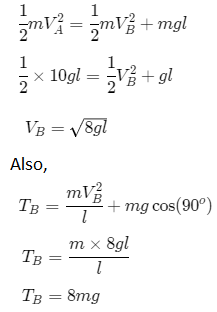(b) Again, from law of conservation of energy:

EA = EC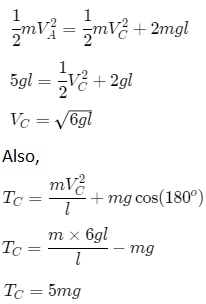(c) Again, from law of conservation of energy:

EA = ED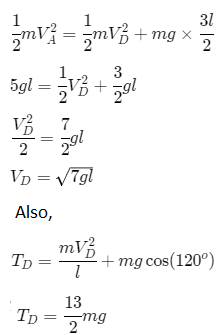Question 54: A simple pendulum consists of a 50 cm long string connected to a 100 g ball. The ball is pulled aside 60 that the string makes an angle f 37o with the vertical and is then released. Find the tension in the string when the bob is at its lowest position.

Solution:

The length of the bob = 50 cm

Ball is pulled at a distance of 60 cm making the angle of 37o. (Given)

Now, total energy in terms of kinetic and potential energy is,

ET = ( ½) mv2 + mgl

and

T = mv2/r + mg

Let EA be the energy at the point which is lowest in the bob circulation and the position of bob at an angle of 37o, from the normal is given as point EB.

Now, 1/2 mv2 = mg (1 – lcosθ)

Here θ = 370 and l = 0.5

=> (1/2) mv2 = mg(0.5 – 0.4)

or v = 1.414

And tension is, T = mv2/r + mg

= (0.1×2)/0.5 + 10

= 1.4 N

Question 55: Figure (before) shows a smooth track, a part of which is a circle of radius R. A block of mass m is pushed against a spring of spring constant k fixed at the left end and is then released. Find the initial compression of the spring so that the block presses the track with a force mg when it reaches the point P, where the radius of the track is horizontal.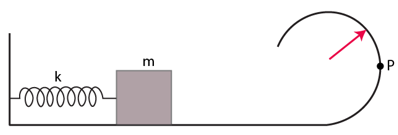Solution:

Using the conservation of static and dynamic energy such as centripetal and kinetic energy, we have the conservation equation, (mv2/r) R = (1/2) kx2

Where, m = mass of the object, v = velocity, k = spring constant and x = elongation distance, R = radius of the circular part, and r = radius of the object.

The energy of the block in form of kinetic energy is equated with the centripetal energy of the surface making the equation

mv2/r = (1/2) kx2 …(1)

Using v = gR

Putting value of v in (1), we have

x = √(3mgR/k)

Question 56: The bob of a stationary pendulum is given a sharp hit to impart it a horizontal speed of √(3gl) Find the angle rotated by the string before it becomes slack.

Solution:

V = √(3gl) … (Given)

(½) mv2 – (1/2) mu2 = -mgh

or v2 = 3gl – 2gl(1 + cosθ) …(i)

Again, mv2/l = mg cos θ

or v2 = lg cos θ …(ii)

From (i) and (ii), we have

θ = cos-1 (1/3)

Thus, angle rotating before the string become slack

θ = 180o – cos-1 (1/3)

=> angle reached before slack = θ = cos-1 (1/3)

Question 57: A heavy particle is suspended by a 15 m long string. It is given a horizontal velocity of √57 m/s. (a) Find the angle made by the string with the upward vertical, when it becomes slack. (b) Find the speed of the particle at this instant. (c) Find the maximum height reach by the particle over the point of suspension. Take g = 10 m/s2.

Solution:

The length string = 15 cm

Horizontal velocity of √57 m/s

Acceleration due to gravity = g = 10 m/s2.

Total energy in terms of kinetic and potential energy:

ET = (1/2) mv2 + mgl

and

Tension = T = mv2/r + mg

(a) angle made before it becomes slack

(1/2) mv2 = (1/2) mu2 + mgh

or v2 = u2 + gh

We know u2 = 57 and h = -3(1+cos θ)

=> v2 = 57 – 3g(1 + cosθ) ….(1)

Using the equation for the bob in terms of centripetal force in (1) i.e., v2 = lg cosθ

(1)=> lg cosθ = 57 – 3g(1 + cosθ)

or cos θ = 3/5

or θ = cos-1 (3/5) = 53o

(b) speed of the particle present at a particular time

v2 = 57 – 3g(1 + cosθ)

or v = 3 m/s

[On putting values]

(c) string becomes slack after losing velocity making the maximum height, h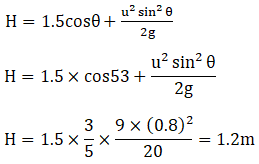Question 58: A simple pendulum of length L having a bob of mass m i deflected from its rest position by an angle θ and released (figure below). The string hits a peg which is fixed at a distance x below the point of suspension and the bob starts going in a circle centred at the peg.

(a) Assuming that initially the bob has a height less than the peg, show that the maximum height reached by the bob equals its initial height.

(b) If the pendulum is released with θ = 90o and x = L/2 find the maximum height reached by the bob above its lowest position before the string becomes slack.

(c) Find the minimum value of x/L for which the bob goes in a complete circle about the peg when the pendulum is released from θ = 90°.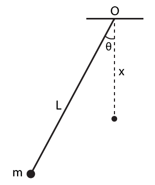Solution:

The pendulum has a mass “m” attached to length “l” which hits the peg hanging at “x” situated at an angle of θ. (Given)

Total energy in terms of kinetic and potential energy:

ET = (1/2) mv2 + mgl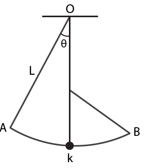(a) When the height of the bob is less than the peg than the total potential energy of the bob at point A is equal to the potential energy of the bob at point B.

K.E. at both places is zero.

P EA = P EB = maximum height of the bob is equivalent to the initial height.

(b)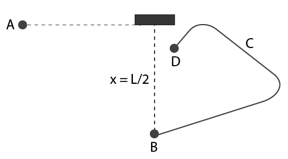When the particle is released at angle θ and x = L/2, the path of the bob travelling will slack at point C making a projectile motion.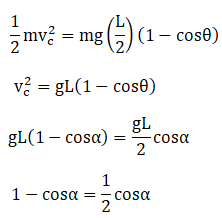Distance after slack at point D:

BD = L/2 + L/2 cosθ = 5/6 L

[When cos θ = 2/3]

(c) The velocity of the bob at point D

vD2 = g(L-x) …(1)

The conservation of the force in the bob is

mg = m vD2/(L-x)

using (1)

mg = mg(L-x)/(L-x)

x/L = 3/5 = 0.6

Question 59: A particle slides on the surface of a fixed smooth sphere starting from the topmost point. Find the angle rotated by the radius through the particle, when it leaves contact with the sphere.

Solution:

The particle moving portrays a centripetal force of mv2/r and a force of mg.

On equating the forces, we have

mg cos θ – N = mv2/r

Find the value of velocity:

mg cosθ = mv2/r

v2 = rg cos θ

and r – r cos θ is the real height.

Let PE be the potential energy due to height is PE = mg(r – r cos θ)

Let us place kinetic and potential energy together, then

g(r – r cos θ) = (1/2) mr cosθ

or 3/2 cos θ = 1

or θ = cos-1(2/3)

Question 60: A particle of mass m is kept on a fixed, smooth sphere of radius R at a position, where the radius through the particle makes an angle of 300 with the vertical. The particle is released from this position. (a) What is the force exerted by the sphere on the particle just after the release? (b) Find the distance travelled by the particle before it leaves contact with the sphere.

Solution:

Using conservation of static and dynamic energy such as potential and centripetal energy, we have the conservation equation as

(mv2/r) R = mgh

(a) The mass of the particle when horizontal

N = mg cos 300 = (√3/2) mg = force exerted by the sphere

(b) The distance travelled by the particle in terms of radian/degree

The change in potential energy due to the angle of 300

mg R cos 300 = R cos(θ + 300)

Let us equate kinetic and potential energy together to find the value of velocity

(1/2) mv2 = mg R cos 30o – R cos(θ + 30 o) and mv2/R = mg cosθ

=> gRcos(θ + 30 o) = 2gR cos 30 o – R cos(θ + 30 o)

On solving above equation, we have

or θ = 25 o or θ = 0.43 radian

Question 61: A particle of mass m is kept on the top of a smooth 8phere of radius R. It is given a sharp impulse which imparts it a horizontal speed v. (a) Find the normal force between the sphere and the particle just after the impulse. (b) What should be the minimum value of v for which the particle does not slip on the sphere? (c) Assuming the velocity v to be half the mininium calculated in part, (d) find the angle made by the radius through the particle with the vertical when it leaves the sphere.

Solution:

The radius of the sphere on which the particle is kept is R and the horizontal speed is taken as v. …(Given)

Using the conservation of static and dynamic energy such as centripetal and potential energy,

(mv2/r) R – N = mgh

(a) When a particle is kept on the top of the sphere a downward force of “mg” and an upward force of “N” is applied on the block giving the equation of forces as

mg – N = mv2/R

the normal force of the particle = N = mg – mv2/R

(b) When a particle is at minimum velocity, the reactionary force becomes zero

mg – N = mv2/R

Here N = 0

=> v = √gR

(c) when velocity as half : v = 1/2 x √gR

=> v2 = (1/4) gR

(d) Find the value of angle

mv’2/R = mg cosθ

Let v’ is the velocity of the particle leaving the sphere

=> 1/2 mv’2 – 1/2 mv2 = mgR(1 – cosθ)

Putting v2 = (1/4) gR and v’ = Rg cosθ

we have,

(1/2) Rg cos2θ – (1/8) gR2 = gR(1 – cos θ)

or θ = cos-1 (3/4)

Question 62: Figure (below) shows a smooth track which consists of a straight inclined part of length l joining smoothly with the circular part. A particle of mass m is projected up the incline from its bottom. (a) Find the minimum projection-speed v0 for which the particle reaches the top of the track. (b) Assuming that the projection-speed is 2v0 and that the block does not lose contact with the track before reaching its top, find the force acting on it when it reaches the top. (c) Assuming that the projection-speed is only slightly greater than v0, where will the block lose contact with the track?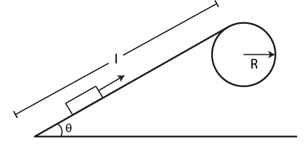Solution:

The formula for the total energy in terms of kinetic and potential energy:

ET = (1/2) mv2 + mgl

(a) height of the particle

H = l sinθ + h

H = l sinθ + (R – R cosθ)

Now, the PE at the top of the sphere

mgH = mg(l sinθ + (R – R cosθ))

Total energy experienced on the particle = T = PE + KE

Initial PE = 0 and initial KE = (1/2) mv20

=> Total energy = (1/2) m v20

Now, equation of total energy, we have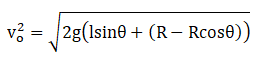(b) If initial speed is 2v0 and final velocity is v

Total energy = PE + KE

(1/2) mv2 + mgH = (1/2)m(2 v20)

v2 = 4v0 – 2gH

Again, centripetal acceleration = v2/R

Therefore, the force acting = F = m/R x (4vo – 2gH)

= (6gR(1-cosθ) + l sinθ)

=> F = 6mg(1 – cos θ + lsinθ/R)

(c) If the speed is doubled

velocity = 2vo

KE = (1/2) mv2

and PE = mg(R – R cos θ)

gR cosθ = 2gR(1 – cosθ)

or 3 cos θ = 2

or θ = cos-1(2/3)

Question 63: A chain of length l and mass m lies on the surface of a smooth sphere of radius R > l with one end tied to the top of the sphere.

(a) Find the gravitational potential energy of the chain with reference level at the centre of the sphere. (b) Suppose the chain is released and slides down the sphere. Find the kinetic energy of the chain, when it has slid through an angle θ.

(c) Find the tangential acceleration dv/dt of the chain when the chain starts sliding down.

Solution:

The formula for the total energy in terms of kinetic and potential energy:

ET = (1/2) mv2 + mgl sinθ

(a) Let α be the angle formed by the chain, and l be the length

Angle can be written as α = l/R

Length of the chain in terms of radius = l = Rdθ

Force derivative of the chain = F = (m/l) R dθ

The potential energy is calculated as

Now, the P.E. = (mR2g/l) cosθ dθ

The PE after integration = (mR2g/l) sin(l/R)

(b) On equating kinetic energy and the potential energy of the chain, we have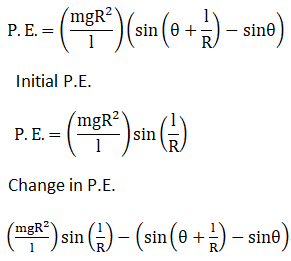(c) find the tangential velocity

on differentiating u with respect to t, we have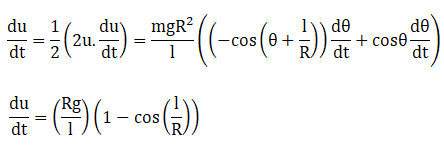Question 64: A smooth sphere of radius R is made to translate in a straight line with a constant acceleration α. A particle kept on the top of the sphere is released from there at zero velocity with respect to the sphere. Find the speed of the particle with respect to the sphere as a function of the angle θ it slides.

Solution: The radius of the smooth sphere is R, the constant acceleration is α.

A force f acts on the sphere towards left, so the particle experience pseudo force = f = ma towards right.

Initial kinetic energy of the particle = zero.

Let the speed of the particle at point C be v.

From work-energy theorem, Wg + Wf = ΔK.E. = K.E.f

Where Wg = work done by the gravity and Wf= work done by pseudo force.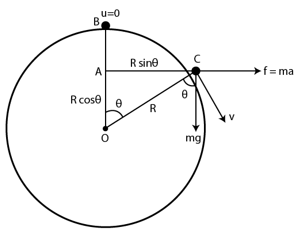From figure, AB = R − Rcosθ = R(1−cosθ). Also, AC = Rsinθ

mgR(1 – cosθ) + ma R sinθ = (1/2) mv2

at a = g

mgR(1 + sin θ – cosθ) = (1/2) mv2

or